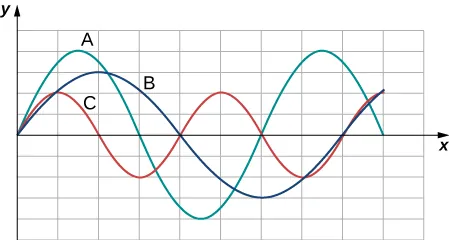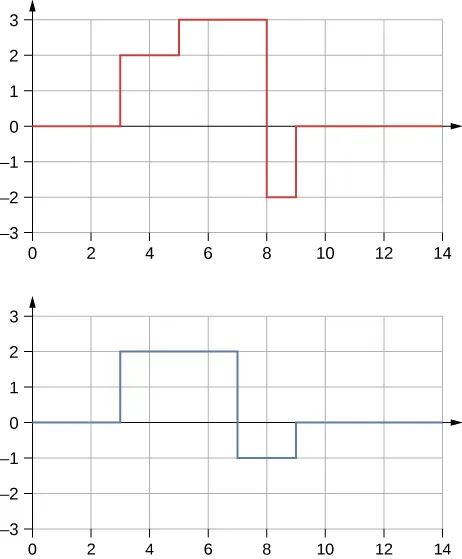University Physics Volume 1

# Conceptual Questions

University Physics Volume 1Conceptual Questions

### 16.1Traveling Waves

1.

Give one example of a transverse wave and one example of a longitudinal wave, being careful to note the relative directions of the disturbance and wave propagation in each.

2.

A sinusoidal transverse wave has a wavelength of 2.80 m. It takes 0.10 s for a portion of the string at a position x to move from a maximum position of $y=0.03my=0.03m$ to the equilibrium position $y=0.y=0.$ What are the period, frequency, and wave speed of the wave?

3.

What is the difference between propagation speed and the frequency of a mechanical wave? Does one or both affect wavelength? If so, how?

4.

Consider a stretched spring, such as a slinky. The stretched spring can support longitudinal waves and transverse waves. How can you produce transverse waves on the spring? How can you produce longitudinal waves on the spring?

5.

Consider a wave produced on a stretched spring by holding one end and shaking it up and down. Does the wavelength depend on the distance you move your hand up and down?

6.

A sinusoidal, transverse wave is produced on a stretched spring, having a period T. Each section of the spring moves perpendicular to the direction of propagation of the wave, in simple harmonic motion with an amplitude A. Does each section oscillate with the same period as the wave or a different period? If the amplitude of the transverse wave were doubled but the period stays the same, would your answer be the same?

7.

An electromagnetic wave, such as light, does not require a medium. Can you think of an example that would support this claim?

### 16.2Mathematics of Waves

8.

If you were to shake the end of a taut spring up and down 10 times a second, what would be the frequency and the period of the sinusoidal wave produced on the spring?

9.

If you shake the end of a stretched spring up and down with a frequency f, you can produce a sinusoidal, transverse wave propagating down the spring. Does the wave number depend on the frequency you are shaking the spring?

10.

Does the vertical speed of a segment of a horizontal taut string through which a sinusoidal, transverse wave is propagating depend on the wave speed of the transverse wave?

11.

In this section, we have considered waves that move at a constant wave speed. Does the medium accelerate?

12.

If you drop a pebble in a pond you may notice that several concentric ripples are produced, not just a single ripple. Why do you think that is?

### 16.3Wave Speed on a Stretched String

13.

If the tension in a string were increased by a factor of four, by what factor would the wave speed of a wave on the string increase?

14.

Does a sound wave move faster in seawater or fresh water, if both the sea water and fresh water are at the same temperature and the sound wave moves near the surface? $(ρw≈1000kgm3,ρs≈1030kgm3,Bw=2.15×109(ρw≈1000kgm3,ρs≈1030kgm3,Bw=2.15×109$
$Pa,Bs=2.34×109Pa)Pa,Bs=2.34×109Pa)$

15.

Guitars have strings of different linear mass density. If the lowest density string and the highest density string are under the same tension, which string would support waves with the higher wave speed?

16.

Shown below are three waves that were sent down a string at different times. The tension in the string remains constant. (a) Rank the waves from the smallest wavelength to the largest wavelength. (b) Rank the waves from the lowest frequency to the highest frequency.17.

Electrical power lines connected by two utility poles are sometimes heard to hum when driven into oscillation by the wind. The speed of the waves on the power lines depend on the tension. What provides the tension in the power lines?

18.

Two strings, one with a low mass density and one with a high linear density are spliced together. The higher density end is tied to a lab post and a student holds the free end of the low-mass density string. The student gives the string a flip and sends a pulse down the strings. If the tension is the same in both strings, does the pulse travel at the same wave velocity in both strings? If not, where does it travel faster, in the low density string or the high density string?

### 16.4Energy and Power of a Wave

19.

Consider a string with under tension with a constant linear mass density. A sinusoidal wave is produced by some external driving force. If the frequency, keeping the wave amplitude as it was originally, how is the time-averaged power of the wave affected? If the amplitude of the wave is decreased by half, keeping the wave frequency as it was originally, how is the time-averaged power affected? Explain your answer.

20.

Circular water waves decrease in amplitude as they move away from where a rock is dropped. Explain why.

21.

In a transverse wave on a string, the motion of the string is perpendicular to the motion of the wave. If this is so, how is possible to move energy along the length of the string?

22.

The energy from the sun warms the portion of the earth facing the sun during the daylight hours. Why are the North and South Poles cold while the equator is quite warm?

23.

The intensity of a spherical waves decreases as the wave moves away from the source. If the intensity of the wave at the source is $I0,I0,$ how far from the source will the intensity decrease by a factor of nine?

### 16.5Interference of Waves

24.

An incident sinusoidal wave is sent along a string that is fixed to the wall with a wave speed of v. The wave reflects off the end of the string. Describe the reflected wave.

25.

A string of a length of 2.00 m with a linear mass density of $μ=0.006kg/mμ=0.006kg/m$ is attached to the end of a 2.00-m-long string with a linear mass density of $μ=0.012kg/m.μ=0.012kg/m.$ The free end of the higher-density string is fixed to the wall, and a student holds the free end of the low-density string, keeping the tension constant in both strings. The student sends a pulse down the string. Describe what happens at the interface between the two strings.

26.

A long, tight spring is held by two students, one student holding each end. Each student gives the end a flip sending one wavelength of a sinusoidal wave down the spring in opposite directions. When the waves meet in the middle, what does the wave look like?

27.

Many of the topics discussed in this chapter are useful beyond the topics of mechanical waves. It is hard to conceive of a mechanical wave with sharp corners, but you could encounter such a wave form in your digital electronics class, as shown below. This could be a signal from a device known as an analog to digital converter, in which a continuous voltage signal is converted into a discrete signal or a digital recording of sound. What is the result of the superposition of the two signals?28.

A string of a constant linear mass density is held taut by two students, each holding one end. The tension in the string is constant. The students each send waves down the string by wiggling the string. (a) Is it possible for the waves to have different wave speeds? (b) Is it possible for the waves to have different frequencies? (c) Is it possible for the waves to have different wavelengths?

### 16.6Standing Waves and Resonance

29.

A truck manufacturer finds that a strut in the engine is failing prematurely. A sound engineer determines that the strut resonates at the frequency of the engine and suspects that this could be the problem. What are two possible characteristics of the strut can be modified to correct the problem?

30.

Why do roofs of gymnasiums and churches seem to fail more than family homes when an earthquake occurs?

31.

Wine glasses can be set into resonance by moistening your finger and rubbing it around the rim of the glass. Why?

32.

Air conditioning units are sometimes placed on the roof of homes in the city. Occasionally, the air conditioners cause an undesirable hum throughout the upper floors of the homes. Why does this happen? What can be done to reduce the hum?

33.

Consider a standing wave modeled as $y(x,t)=4.00cmsin(3m−1x)cos(4s−1t).y(x,t)=4.00cmsin(3m−1x)cos(4s−1t).$ Is there a node or an antinode at $x=0.00m?x=0.00m?$ What about a standing wave modeled as $y(x,t)=4.00cmsin(3m−1x+π2)cos(4s−1t)?y(x,t)=4.00cmsin(3m−1x+π2)cos(4s−1t)?$ Is there a node or an antinode at the $x=0.00mx=0.00m$ position?

Order a print copy

As an Amazon Associate we earn from qualifying purchases.